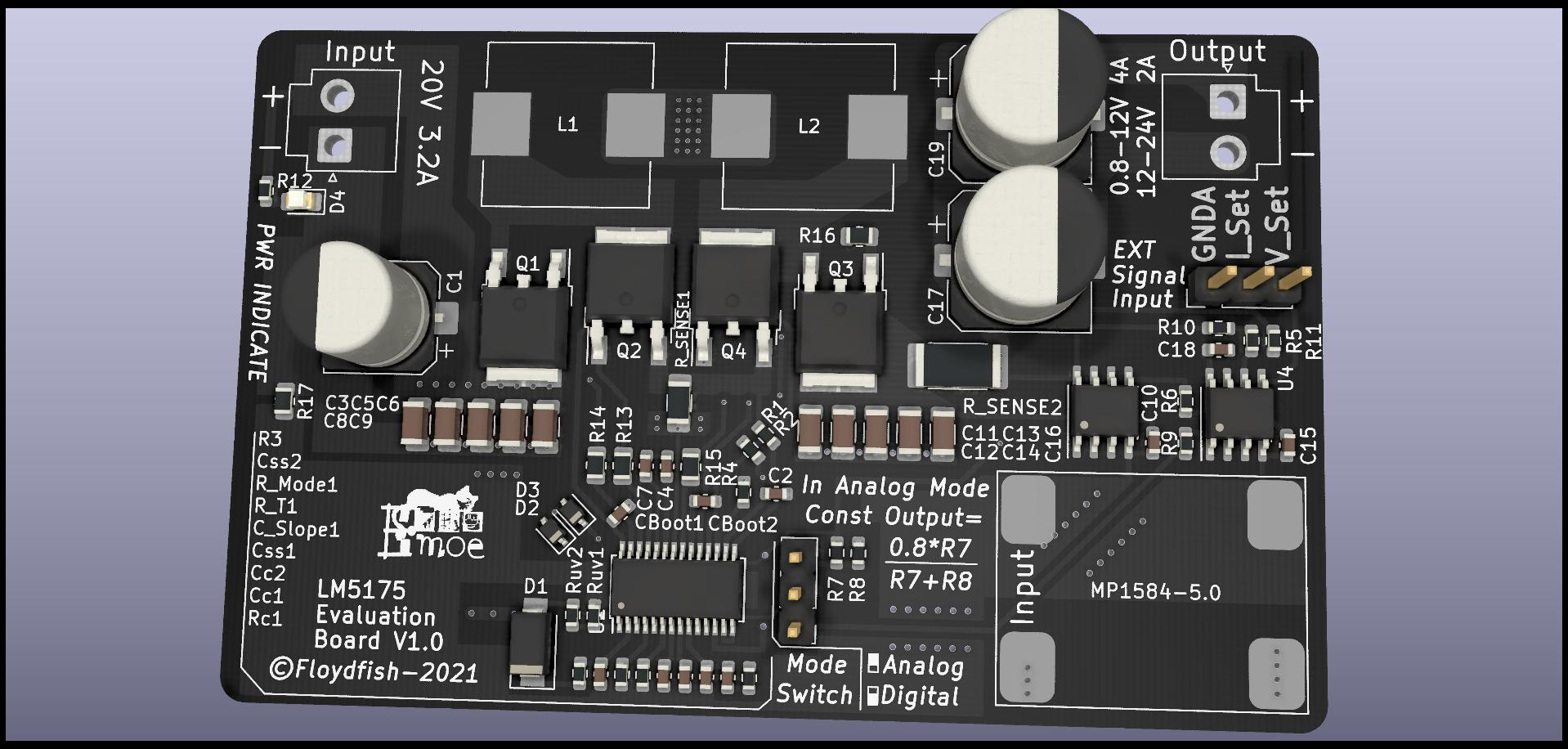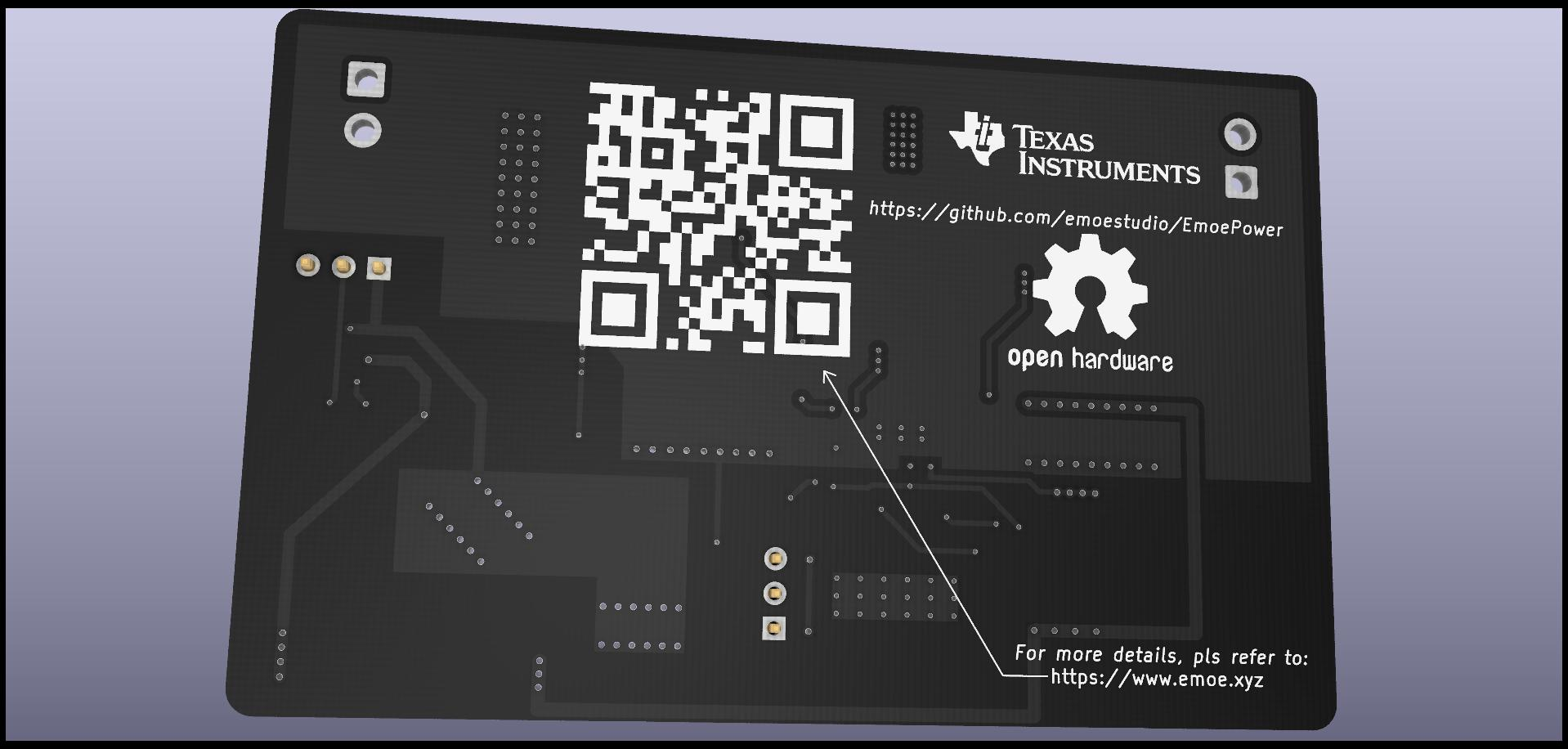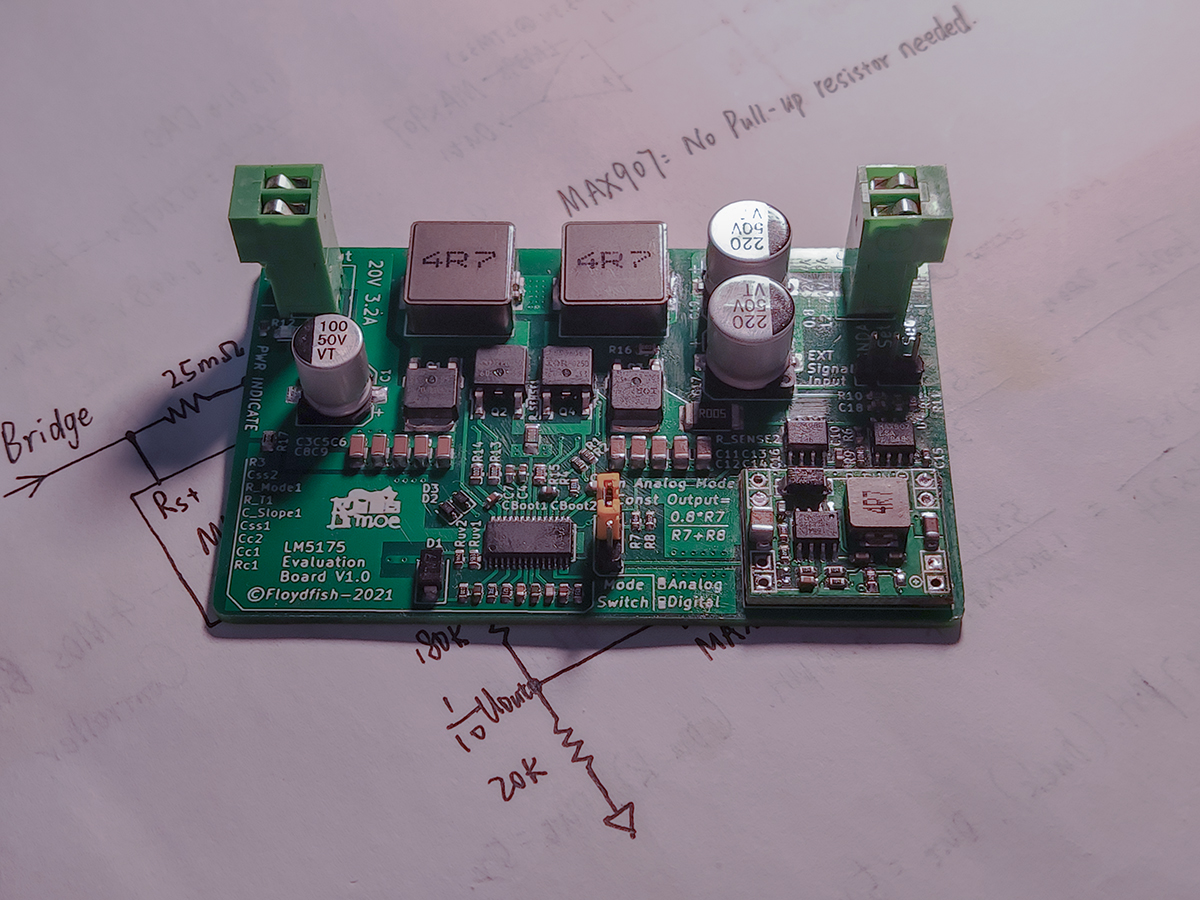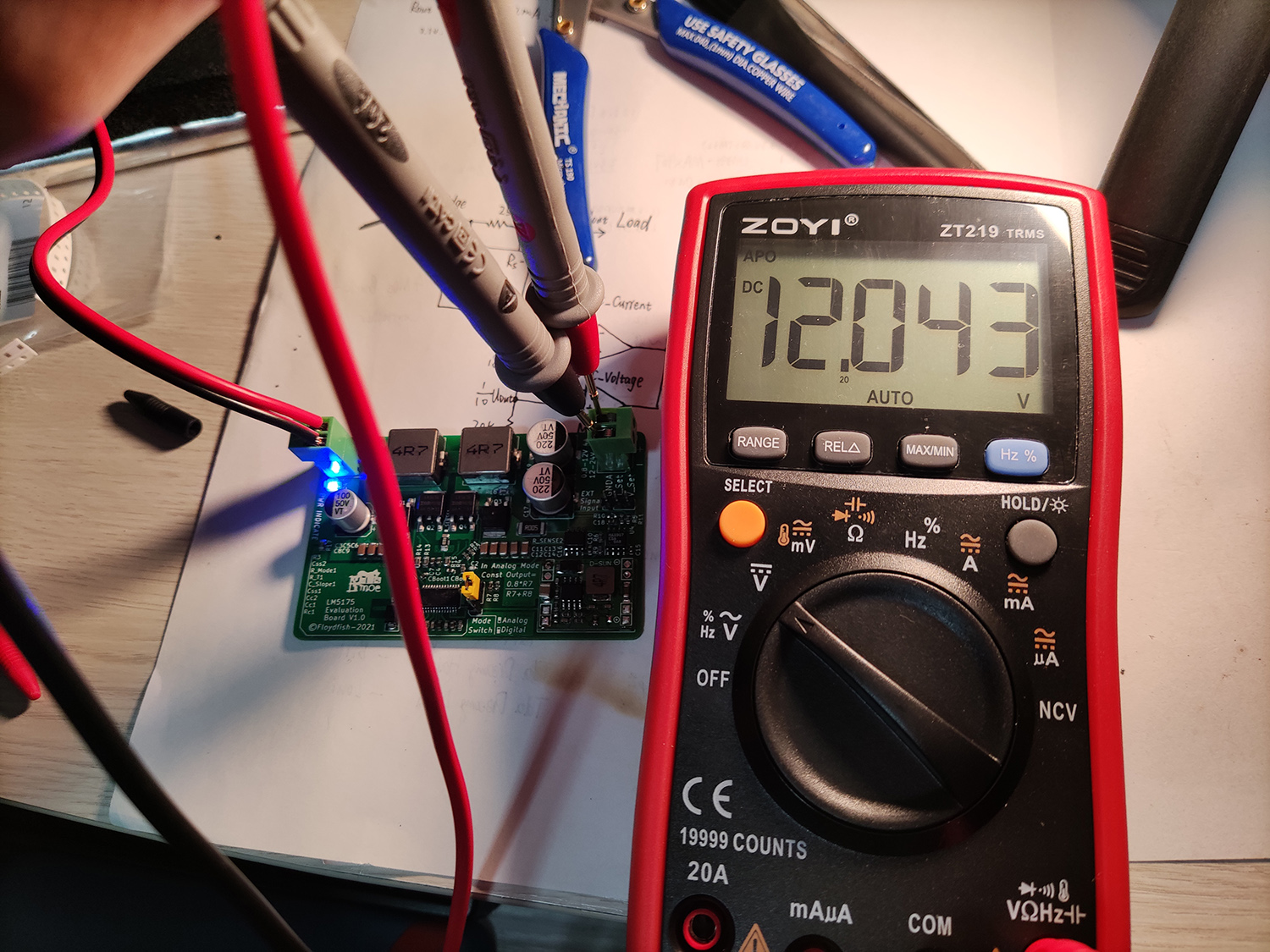# 确定需求

• 小体积
• 可设定输出电压和限流
• 高效率、高可靠性、高精度(三高实锤)
• 多种控制方法(旋转编码器+Wi-Fi远程控制)
• 现代化的GUI(还要好看才行~)
• (Optional)使用锂电池组作为内置能源,可离线使用
• (Optional)支持多设备级联,支持并联/串联同步调节
• (Optional)做一个精致的外壳~

# 开工——V1.0版本

LM5175工作模式 CCM & Hiccup On

## PCB Design

tip:

LM5175的数据手册里就有这样的例程，我也参考数据手册的例程进行了布局和布线。## LM5175参数简单计算

### Frequency Calc

R_T={{{1 \over F_{SW}}-200ns}\over{37pF}}

Finally get Rt = 84.5kΩ and we select standard value, that is 82kΩ.

### Inductor Selection

L_{BUCK}={({{V_{IN(MAX)}-V_{OUT})*V_{OUT}}}\over{0.4*I_{OUT(MAX)}*F_{SW}*V_{IN(MAX)}}}

In most cases, circuit works in BUCK mode, so choose a most-common used voltage range, and calculate its L, finally we get L is about 10uH.

And the maximum average inductor current occurs at the minimum input and maximum load current:

I_{L(MAX)}={{V_{OUT}*I_{OUT(MAX)}}\over{0.9*V_{IN(MIN)}}}
I_{L(PEAK)}=I_{L(MAX)}+{{V_{IN(MIN)}*(V_{OUT}-V_{IN(MIN)})}\over{2*L_1*F_{SW}*V_{OUT}}}

Finally we choose two 1265 4.7uH in series.

### What’s left…

1.2.